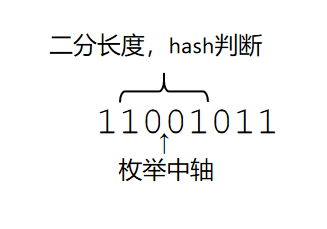# LOJ#2452. 「POI2010」反对称 Antisymmetry

48分做法

100分做法#include<iostream>
#include<cstdio>
#include<algorithm>
#include<cstring>
using namespace std;
typedef unsigned long long ull;
typedef long long ll;
const int N=5e5,base=1e9+7;
char s[N+10];
ull h1[N+10],h2[N+10],p[N+10];
bool t1[N+10],t2[N+10];
int n;
void init()
{
p=1ull;
for(int i=1;i<=n;i++) p[i]=p[i-1]*base;
for(int i=1;i<=n;i++) h1[i]=h1[i-1]*base+(s[i]=='1');
for(int i=1;i<=n;i++) h2[i]=h2[i-1]*base+(s[n-i+1]=='0');
}
ull hs(ull *h,int l,int r) {return h[r]-h[l-1]*p[r-l+1];}
bool check(int l,int r) {return hs(h1,l,r)==hs(h2,n-r+1,n-l+1);}
int erfen(int i)
{
int l=0,r=min(i,n-i),ans=0;
while(l<=r)
{
int mid=(l+r)/2;
if(check(i-mid+1,i+mid))
{
l=mid+1;
ans=mid;
}
else r=mid-1;
}
return ans;
}
int main()
{
ll ans=0;
scanf("%d%s",&n,s+1);
init();
for(int i=1;i<n;i++) ans+=erfen(i);
printf("%lld",ans);
return 0;
}


posted @ 2020-07-12 22:39  zzt1208  阅读(34)  评论(2编辑  收藏